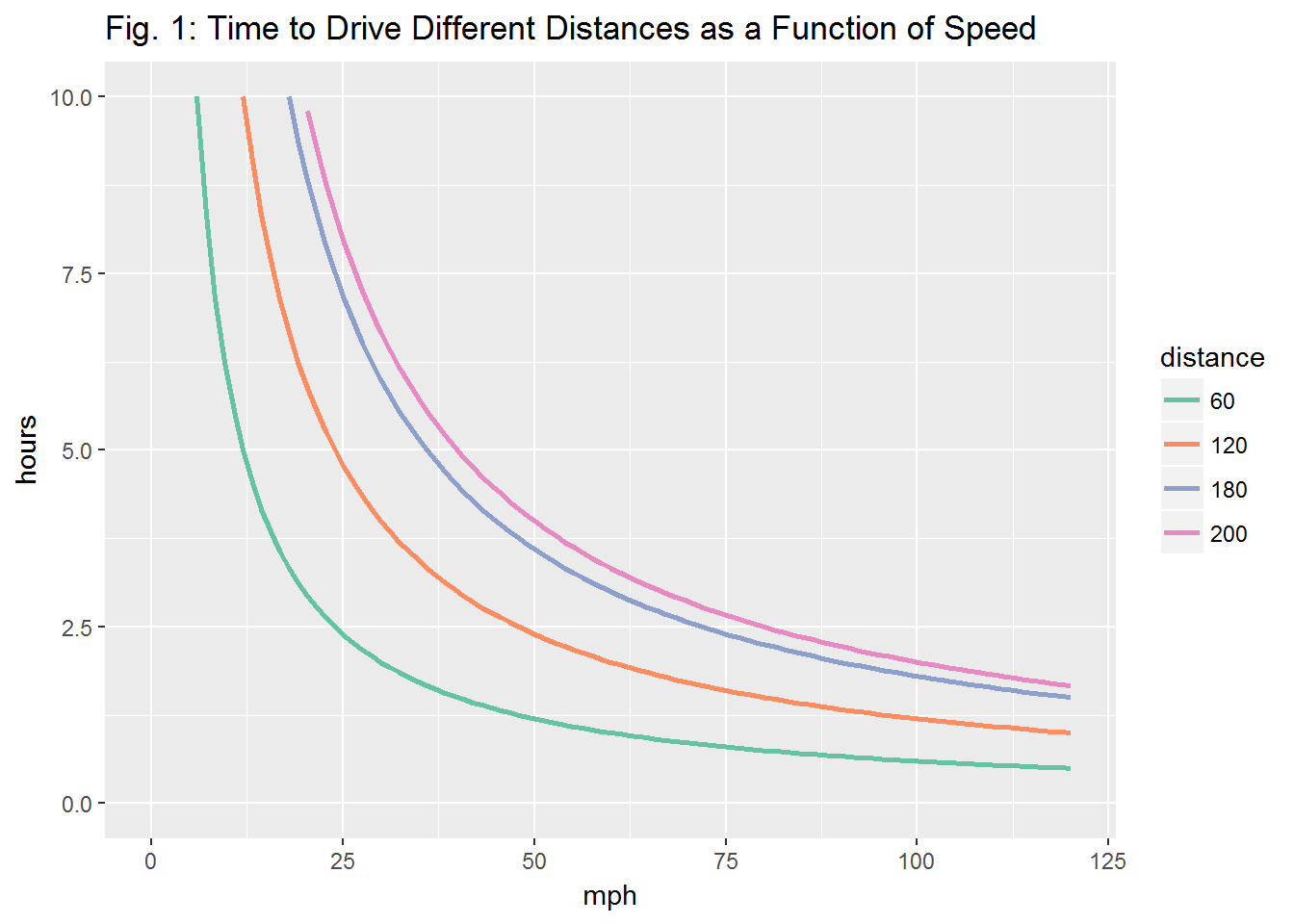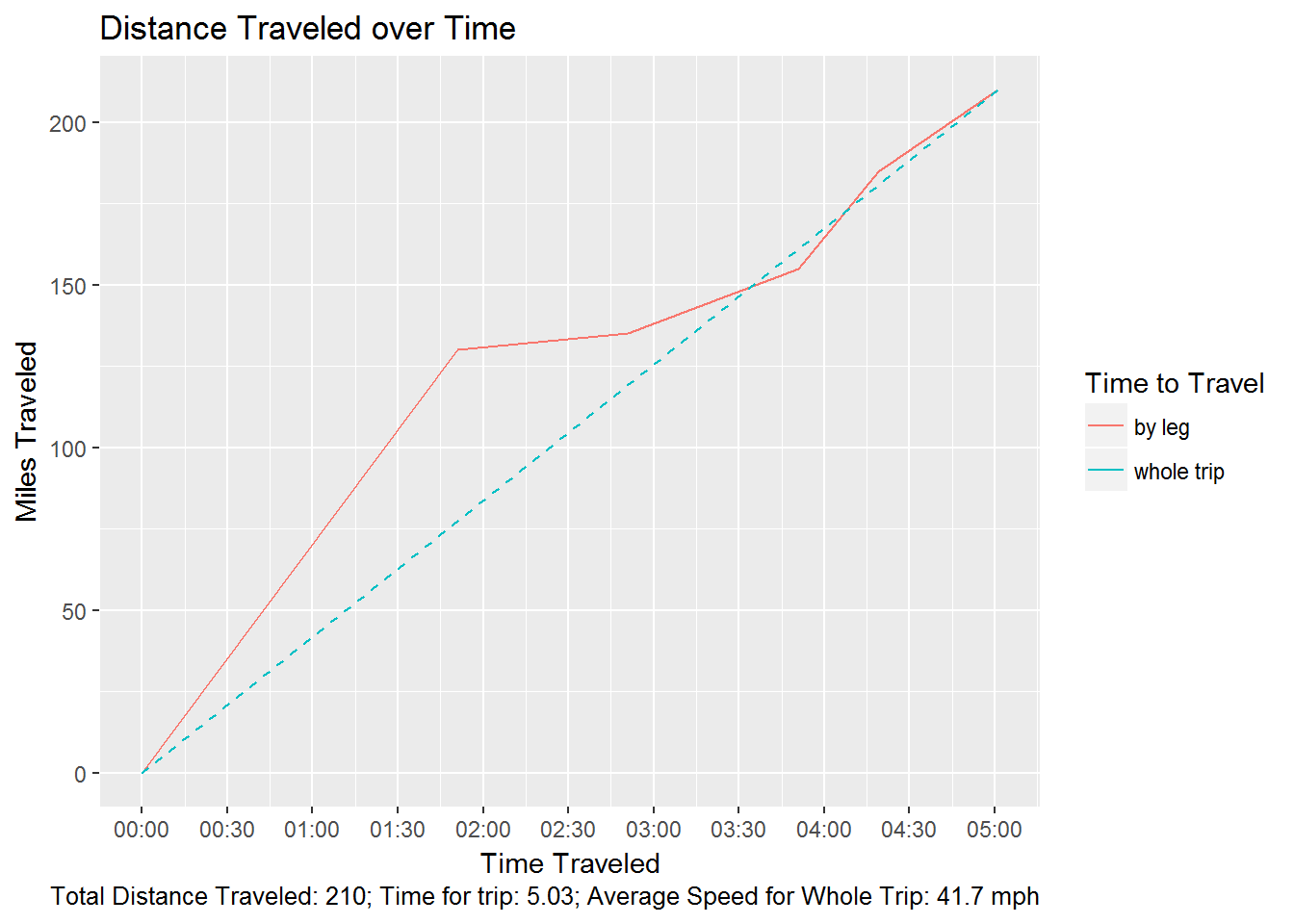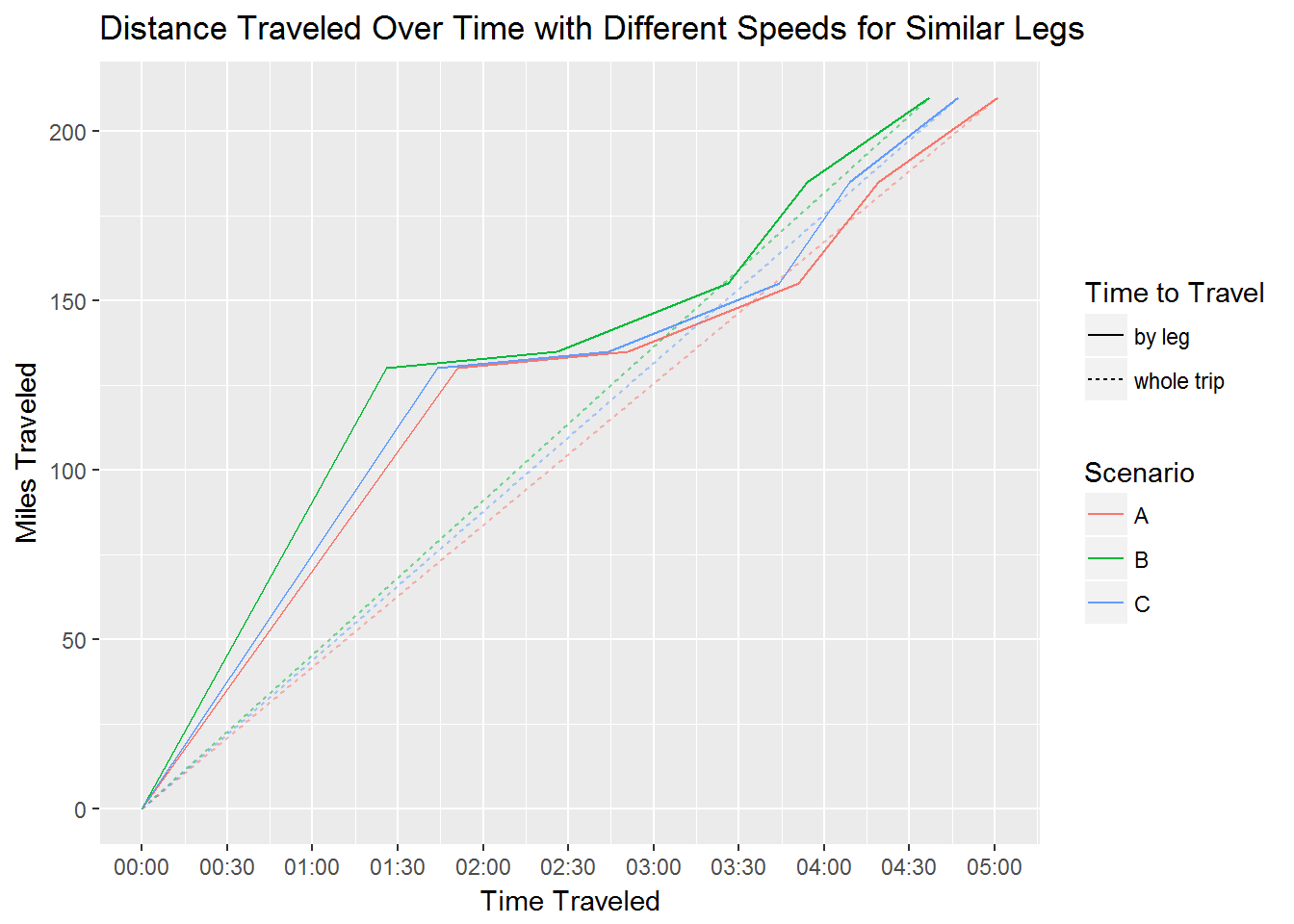## Posing the Question

I’m a nerd. I think that’s pretty obvious from this blog. One thing I do to stay awake on long drives at night is to do math problems in my head; a particular favorite of mine is to calculate how fast I need to drive to arrive at my destination half an hour earlier. The answer is surprisingly bleak: speeding really doesn’t save that much time. But calculating various contingencies and corollaries in my head does tend to keep me awake for 10-15 minutes, so there’s that.

## Question 1: How Much Time does Speeding Save?

Short answer? Not as much as you might think. The longer answer is that the relationship between travel time and speed is an inverse one: $$time=\frac{distance}{speed}$$. You may remember a similar inverse equation from math class: $$y=\frac{1}{x}$$. This is a curve that never quite touches either axis going from $$x=0$$ out to $$x\Rightarrow\infty$$. So we can plot time on the y-axis as a function of speed for a variety of different distances to get a graphic for how long a trip will take at different average speeds: Figure 1.We can pretty quickly see that at low speeds, going one mile per hour faster will have a pretty sizable impact on total time. However, by the time we reach the highway speed limit (65, 70, or 75, depending on the state), A one mph increase is going make a pretty small change in total change: not zero, but not necessarily substantial. For a better view of this in terms of minutes saved, here’s one of the above lines in table format:

Speed Hours Time
40 1.5000 01:30:00
50 1.2000 01:11:01
60 1.0000 01:00:00
70 0.8571 00:51:00
80 0.7500 00:45:00
90 0.6667 00:40:00

Going from 40 mph to 50 mph will save 19 minutes, but going from 80 mph to 90 mph will only save 5 minutes: Same 10 mph increase, but pretty big differences in time saved. More formally, an increase in speed of X% means a $$\frac{1}{1+\frac{X}{100}}%$$ decrease.

• $$OldTime=\frac{Distance}{OldSpeed}$$
• $$NewSpeed = (1+\frac{X}{100}) * OldSpeed$$
• $$NewTime = \frac{Distance}{NewSpeed}$$
• $$NewTime = \frac{Distance}{(1+\frac{X}{100}) OldSpeed}$$
• $$NewTime = (\frac{1}{(1+\frac{X}{100}})(\frac{Distance}{OldSpeed})$$
• $$NewTime = \frac{OldTime}{1+\frac{X}{100}}$$

The above formula might look complicated, but when put in fraction form rather than percentage form, it’s just a simple reciprocal (assuming the percentage change makes a nice fraction). In fraction form, If my speed increases by 20% (or one-fifth), that means my new speed is $$\frac{6}{5}$$ of my old speed and my time changes by the reciprocal: $$\frac{5}{6}$$. So a 20% increase in speed means only a 16% decrease in time.

## Question 2: How does speeding for one leg affect the overall average speed?

So far, I’ve been talking about speed like it’s a single value, but what I’m really talking about is average speed, because we know that you never drive the same speed for the whole trip. More realistically, I leave my house and drive 10 miles at an average of 30 mph until I get on the highway, then I’m driving 70 mph on the highway for 130 miles before I traffic drops down to one lane because of an accident or construction or something like that, so it takes me an hour to go just 5 miles followed by some slow 20 mph driving for 20 miles. After that it’s smooth sailing at 65 mph, but only for 30 miles before I take my exit and drive on regular streets with a 40 mph speed limit for 25 miles until I get to my destination. In other words, my distance traveled over time looks something like this (with my overall average speed plotted as the dotted line).So how big of a difference does it make if I drive 90 mph for that 130 mile stretch where I can actually get going and pass people? How much does that accident and rubber-necking-induced slowness negate the benefits of my speeding during the previous or subsequent legs?

In other words, what if our Distance-Over-Time graph looks like any one of these alternatives?The steeper the leg, the more distance we’re traveling in shorter amount of time; i.e. the faster we’re going. But what do those steeper slopes on the legs mean for steeper slopes of the average speed? Or, more importantly, how much time do I save? If the only difference between Trip A and Trip B is to drive 90 mph instead of 70 mph for that 130 leg.

Trip AvgSpeed TotalDist TotalTime
A 41.72 210 05:01:00
B 45.45 210 04:37:00
Diff 3.73 0 00:24:00
• $$TotalTime = \sum\frac{Distance_{leg}}{AvgSpeed_{leg}}$$
• $$AverageSpeed_A=\frac{220}{\frac{10}{30}+\frac{130}{70}+\frac{5}{5}+\frac{20}{20}+\frac{30}{65}+\frac{25}{35}}$$
• $$AverageSpeed_B=\frac{220}{\frac{10}{30}+\frac{130}{90}+\frac{5}{5}+\frac{20}{20}+\frac{30}{65}+\frac{25}{35}}$$

In other words, speed follows a weighted harmonic average, and that addition in the denominator means we don’t have a nice ratio like we did before. In this case, we have a 8.3% increase from 41 mph to 44.4 mph. $$1-\frac{1}{1.083}=0.077$$ so we have a 7.7% decrease in total time. (Yes,$$\frac{24 minutes}{321 minutes} \approx 7.5%$$ but when not parsing this into minutes the math works out.) See Github for details about the below function.

A = funcList$A$totals ; A
##   distance    hours
## 1      210 5.032967
B = funcList$B$totals ; B
##   distance    hours
## 1      210 4.620269
((A-B)/A)\$hours
##  0.08199903

So it doesn’t take much for a large increase in speed, even for a long time, to get washed away by tough traffic or just a long, non-highway stretch through sideroads.

## Some Benefits of Speeding

So speeding, even speeding a lot, doesn’t change the total travel time by a whole lot. Obviously, speeding bears the risk of getting pulled over and getting a ticket, as well as increased risk of accident, and burning more gas. On the other hand, there are some non-mathematical benefits. First of all, it’s fun; plain and simple. Second, I really think speeding does make me more alert while driving since the fun, danger, and adrenaline of speeding makes it easier to focus on the road (and watch for cops) without drifting off into my thoughts, though that is, admittedly, a somewhat dubious and unsupported claim.

## Conclusion

Speeding probably isn’t worth it. The math just doesn’t work out. So the next time someone responds to your question of “how long does it take to drive there?” with the question “do you speed?” Just point them to this blog post as you explain to them that your speeding habits really aren’t relevant to your question.